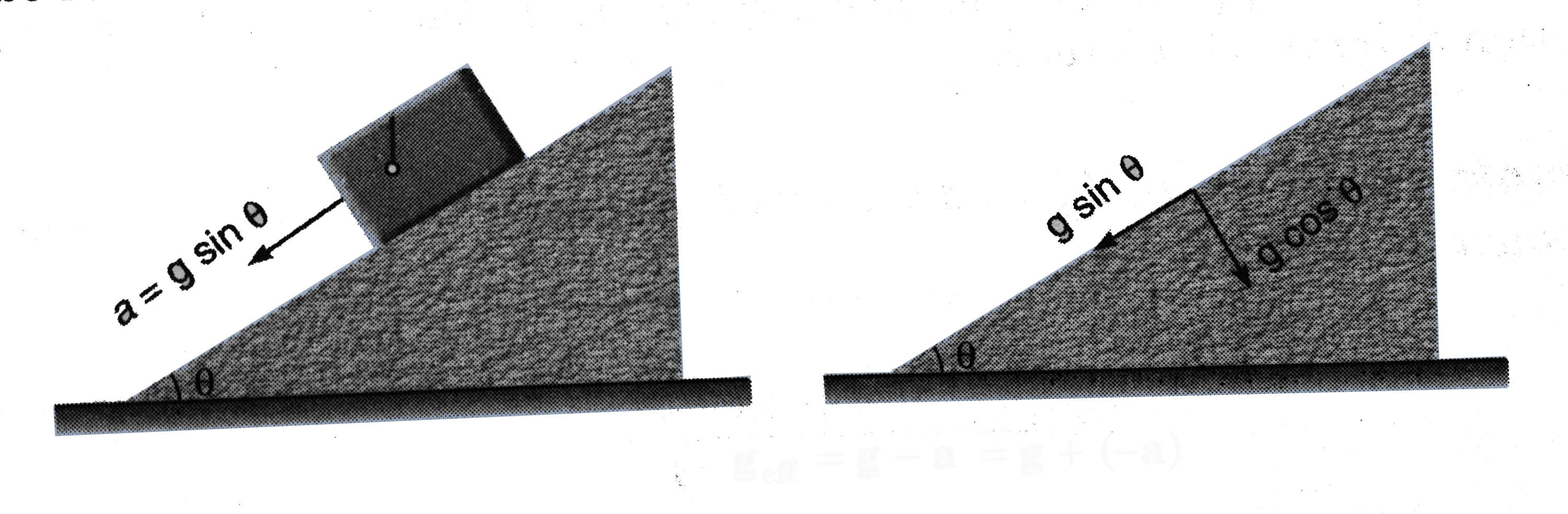# A simple pendulum of length l is suspended from the celing of a cart which is sliding without friction on as inclined plane of inclination theta . W

29 views
in Physics
closed
A simple pendulum of length l is suspended from the celing of a cart which is sliding without friction on as inclined plane of inclination theta . What will be the time period of the pendulum?

by (67.4k points)
selected

Here, point of suspension has an acceleration. a = g sin theta (down the plane). Further, g can be resolved into two components g sin theta (along the plane) and g cos theta (perpendicular to plane).
:. g_(eff) = g - a = g + ( -a)
Resultant of g and -a will be g cos theta.
:. g_(eff) = g cos theta
(perpendicular to plane)
:. T = 2pi sqrt((l)/(|g_(eff)|)) = 2pi sqrt ((l)/(g cos theta)